# CIE A Level Chemistry复习笔记1.5.5 Hess’s Law

### Hess Cycles

• Hess’s Law states that:"The total enthalpy change in a chemical reaction is independent of the route by which the chemical reaction takes place as long as the initial and final conditions are the same."
• This means that whether the reaction takes place in one or two steps, the total enthalpy change of the reaction will still be the same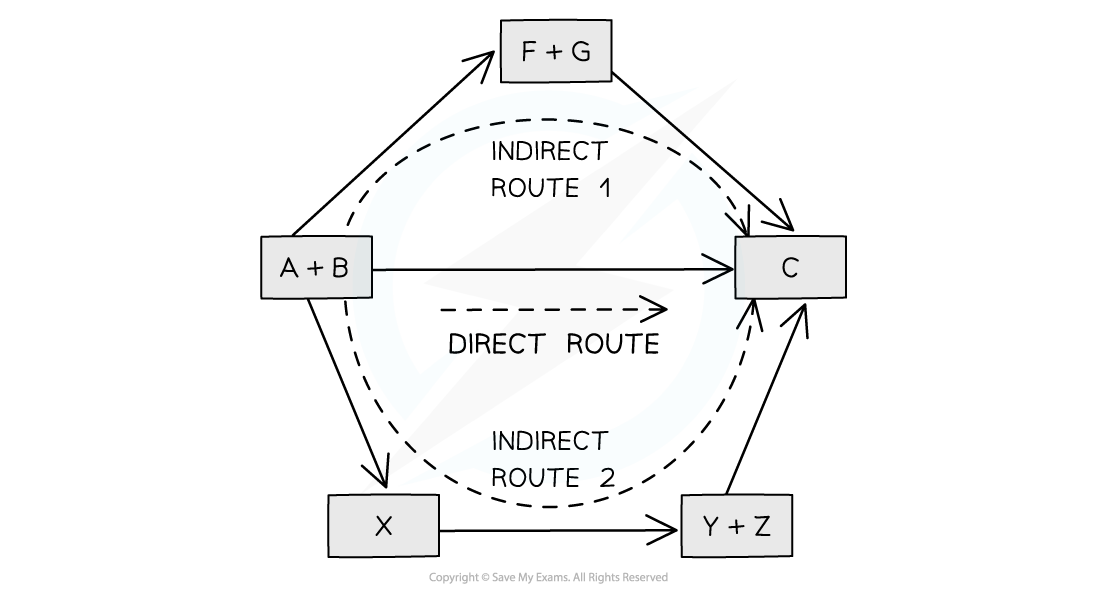The diagram above illustrates Hess’ Law: the enthalpy change of the direct route, going from reactants (A+B) to product (C) is equal to the enthalpy change of the indirect routes

• Hess’ Law is used to calculate enthalpy changes which can’t be found experimentally using calorimetry, eg:

3C (s) + 4H2 (g) → C3H8(g)

• ΔHf (propane) can’t be found experimentally as hydrogen and carbon don’t react under standard conditions

#### Calculating ΔHr from ΔHf using Hess’s Law energy cycles

• The products can be directly formed from the elements = ΔH2

OR

• The products can be indirectly formed from the elements = ΔH1 + ΔHrThe enthalpy change from elements to products (direct route) is equal to the enthalpy change of elements forming reactants and then products (indirect route)

• Equation

ΔH2 = ΔH1 + ΔHr

Therefore,

ΔHr = ΔH2 – ΔH1

#### Worked example: Calculating the enthalpy change of reaction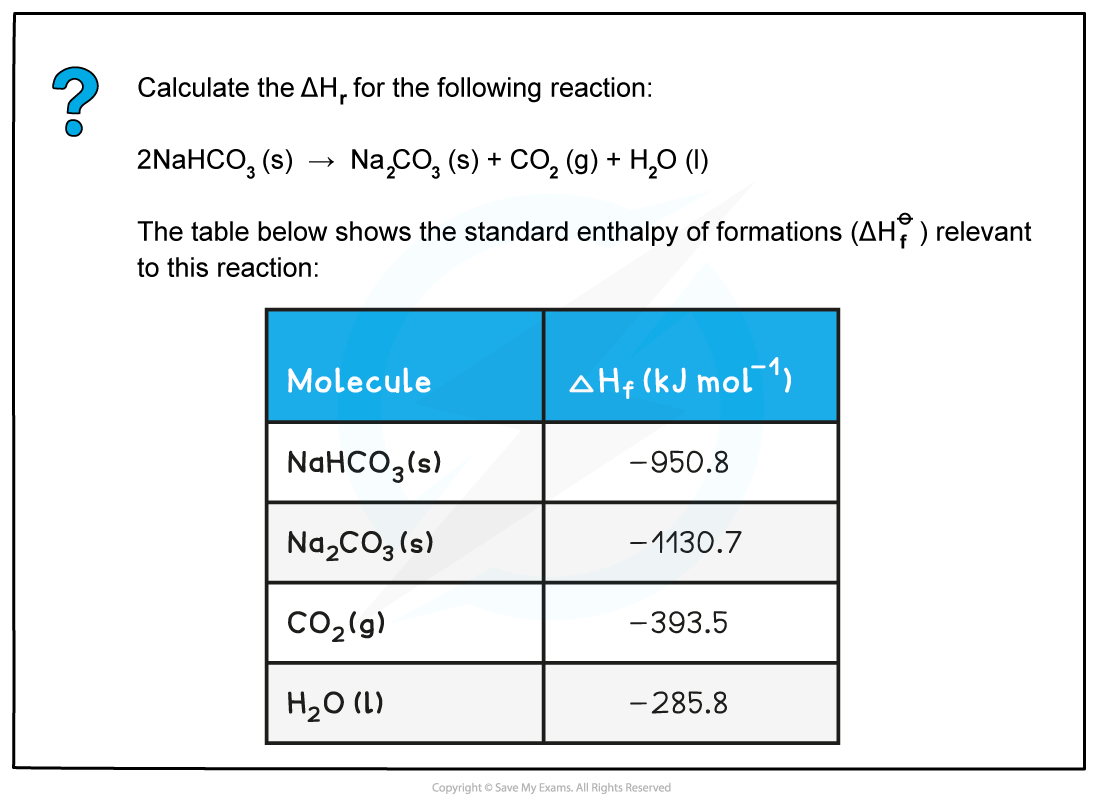• Step 1: Write the balanced equation at the top• Step 2: Draw the cycle with the elements at the bottom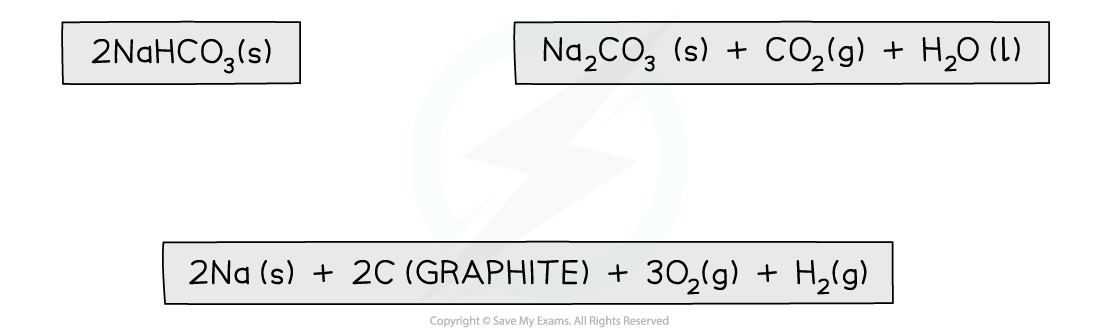• Step 3: Draw in all arrows, making sure they go in the correct directions. Write the standard enthalpy of formations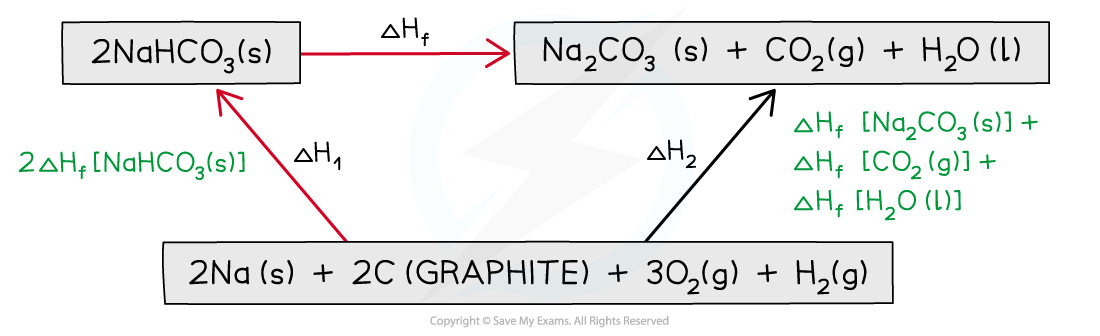Step 4: Apply Hess’s Law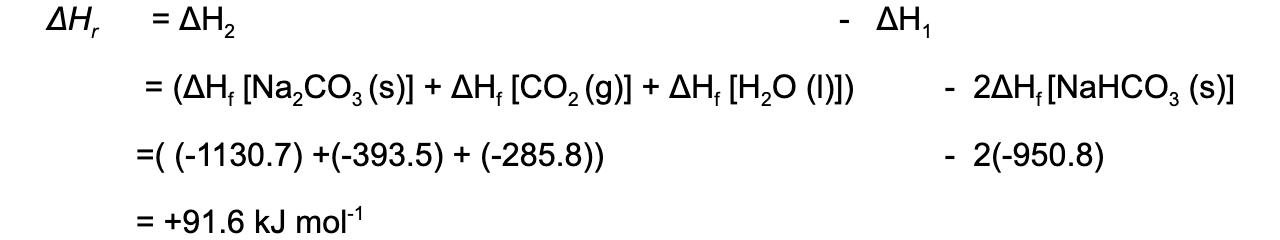#### Calculating ΔHf  from ΔHc using Hess’s Law energy cycles

• The combustion products can be formed directly from elements to combustion products = ΔH1

OR

• The combustion products can be formed indirectly from elements to compound to combustion products = ΔHf+ ΔH2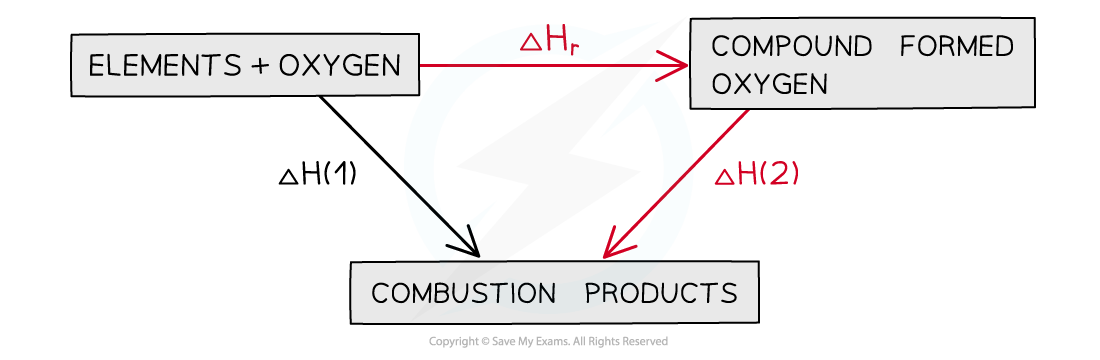The enthalpy change going from elements to products (direct route) is equal to the enthalpy change of elements forming reactants and then products (indirect route)

• Equation

ΔH1 = ΔHf + ΔH2

Therefore,

ΔHf  = ΔH1 – ΔH2

#### Worked example: Calculating the enthalpy change of formation of ethane• Step 1: Write the equation for enthalpy change of formation at the top and add oxygen on both sides• Step 2: Draw the cycle with the combustion products at the bottom• Step 3: Draw all arrows in the correct direction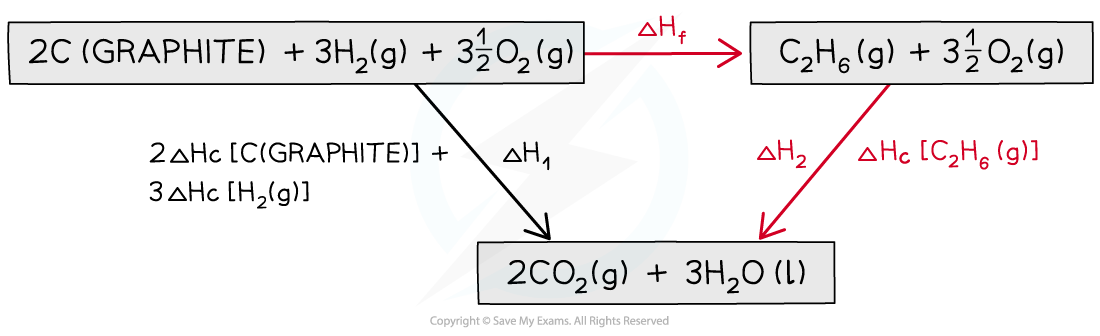• Step 4: Apply Hess’s Law#### Calculating average bond energies using Hess's cycles

• Bond energies cannot be found directly so enthalpy cycles are used to find the average bond energy
• This can be done using enthalpy changes of atomisation and combustion or formation
• The enthalpy change of atomisation (ΔHatꝋ ) is the enthalpy change when one mole of gaseous atoms is formed from its elements under standard conditions.
• Eg. ΔHatꝋ [H2] relates to the equation:

½ H2(g) → H(g)

#### Worked example: Calculating average C-H bond energy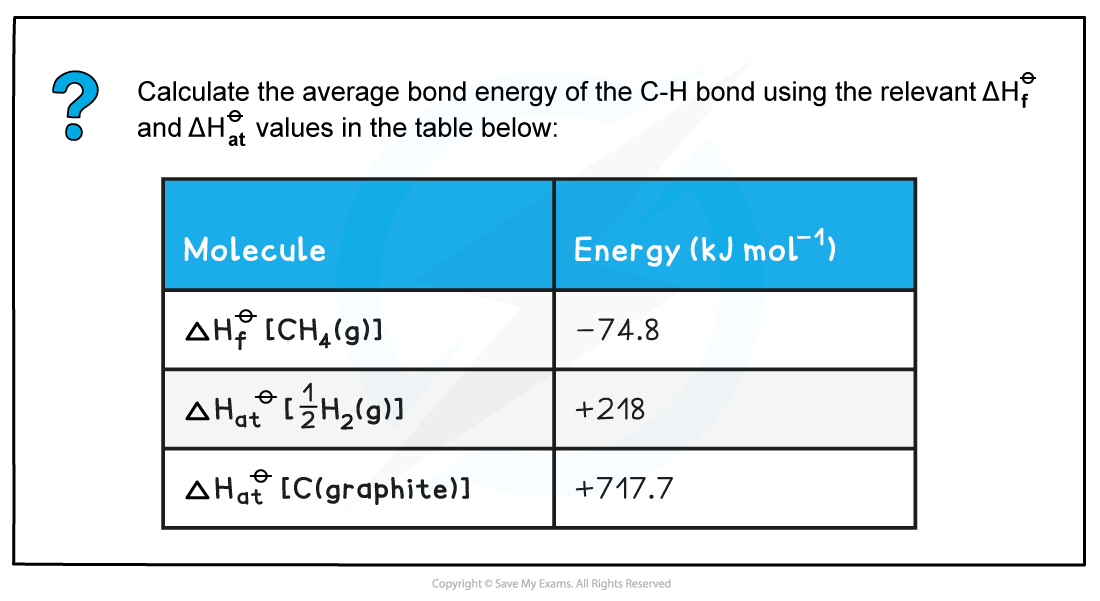• Step 1: Write down the equation for the dissociation of methane at the top• Step 2: Write down the elements at the bottom• Step 3: Draw all arrows in the correct direction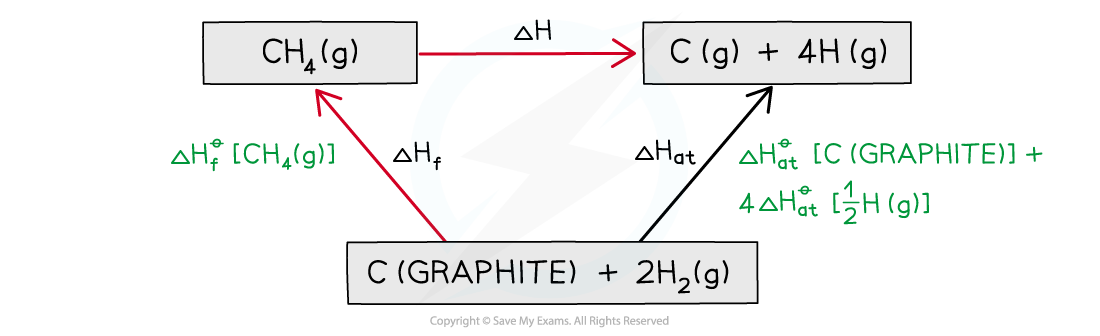• Step 4: Apply Hess’s Law• Step 5: Since there are 4 C-H bonds in methane:#### Exam Tip

Remember to take into account the number of moles of each reactant and product.For example, there are two moles of NaHCO3(s) so the ΔHf value is multiplied by 2.